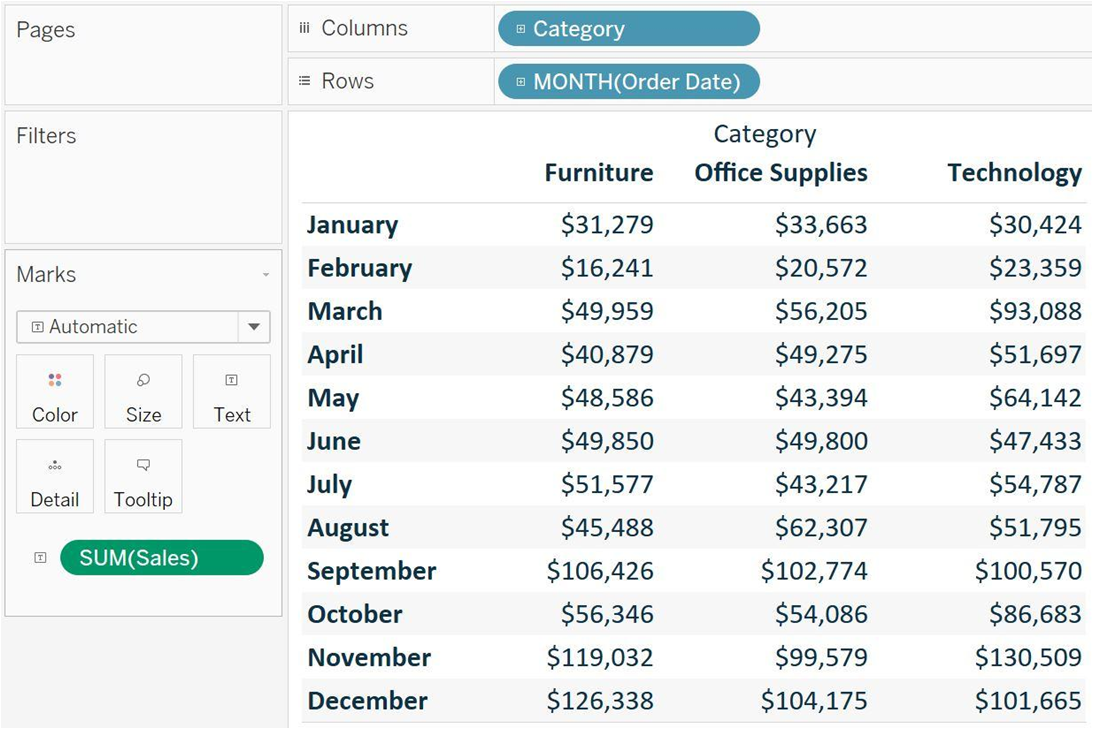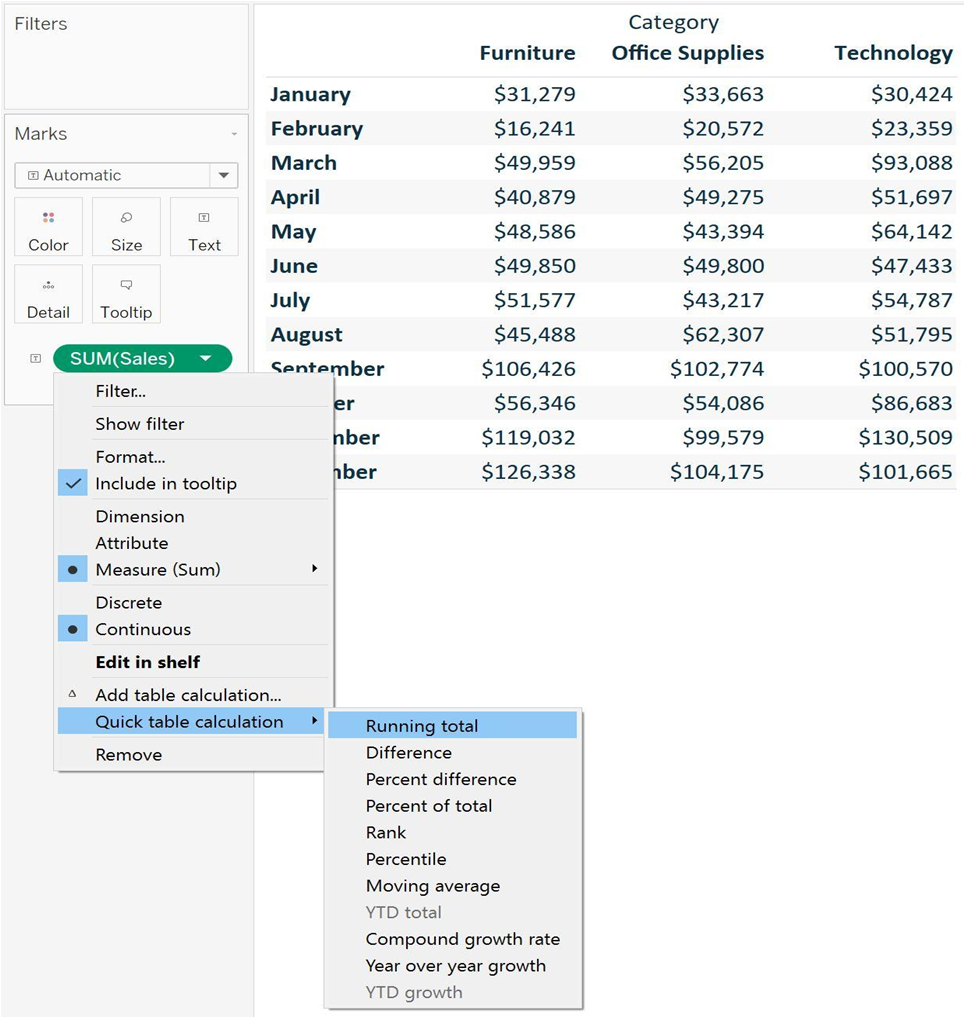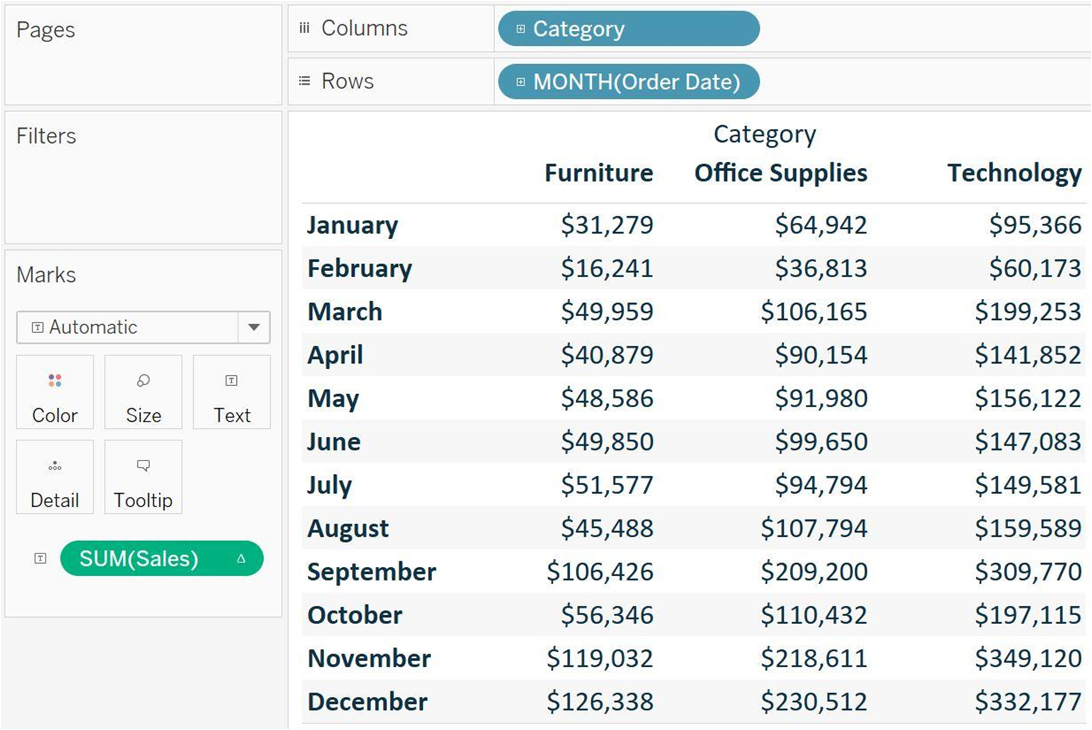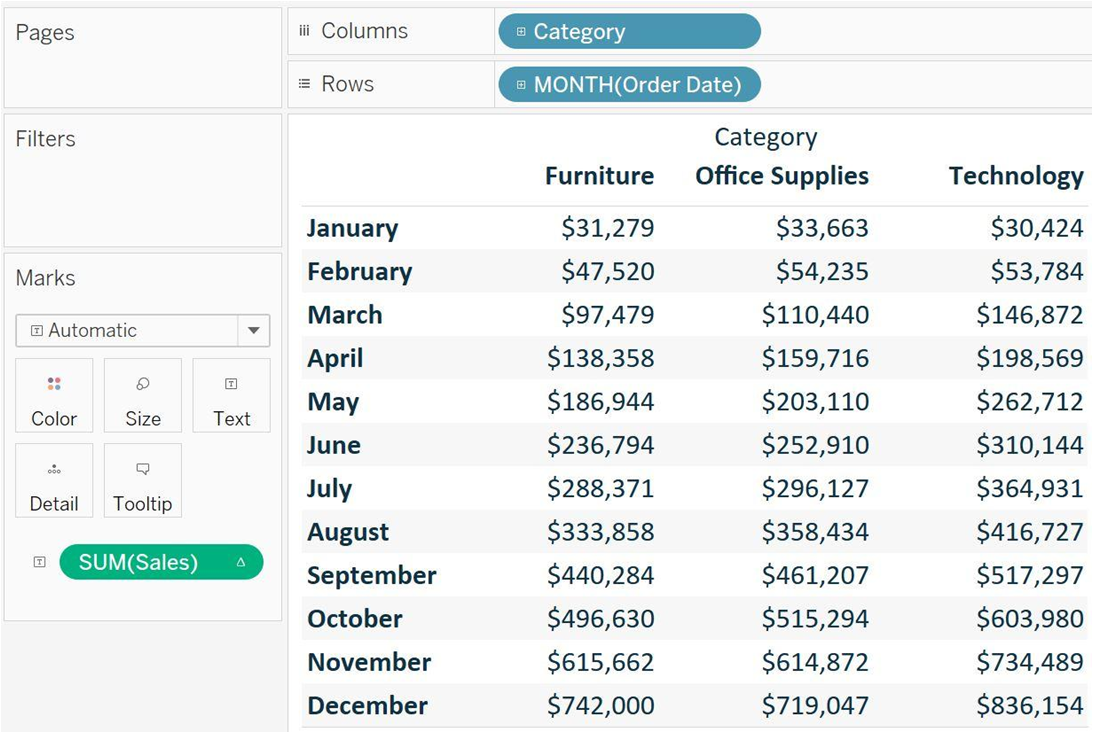# Table calculation in Tableau

Rating: 5

4724

A table calculation is a transformation we apply to the values of a single measure in our view, based on the dimensions in the level of detail.

Want To Get Tableau Training From Experts? Enroll Now For Free Demo On "Tableau Training"

For any Tableau view, there is a virtual table that is determined by the dimensions in the view. This table is not to be confused with the tables in our data source. Specifically, the virtual table is determined by the dimensions within the “level of detail”.

## Tableau Table Calculations

Tableau comes with several preset calculations that you can compute with the numbers on a view including running total, difference, percent difference, percent of the total, moving average, and more. These predefined calculations are called table calculations because they compute the result based on a virtual table that includes only the numbers on the view. Table calculations provide several benefits including:

1. A fast way to create advanced calculations even without knowing the underlying syntax
2. Table calculations can be saved for future use as calculated fields, and because calculated fields 3. can be edited, this is a great way to learn the syntax and the different functions available in Tableau

Efficient processing; table calculations are computed on a very small subset of the data source, making them an efficient solution for calculating results.

Now to see how table calculation works we need to connect to the sample superstore and run an example of calculating the running total.Table calculations are added to measures, so in order to add a table calculation, click a measure that’s on the view. The fastest way to add a table calculation is to hover over “Quick table calculation” and choose an option:After running total, the view will change.Table calculations are defined by how they are (1.) partitioned, and (2.) addressed – or how they are computed. In the example above the running total is being computed from left to right, which is the default address. This would mean that, by default, the table calculation is being addressed by the Product Category dimension. This leaves the Month dimension as the partitioning field.

It is easy to change the addressing by changing how the table calculation is being computed. To do this, click on the measure with the table calculation again, now identified with a delta symbol, hover over compute using, and change how the calculation should be computed.

Now after changing the addressing the view will update.Now that the addressing field has been changed to Month and the partitioning field has been changed to Product Category we can get more sensible analysis to look at each Product Category column, and look down across months to see how the sales built up throughout the year.

Table calculations rely on two types of fields: addressing and partitioning fields. The key to understanding table calculations is to know how these fields work.

Partitioning fields do what it sounds like they do: They partition your data into separate buckets, each of which is acted on by the calculations.

Addressing fields define the “direction” that you want your calculation to take.

Checkout Tableau Interview Questions

### Basic table calculation functions:

LOOKUP(expression, [offset])
This returns the value of the expression in a target row, specified as a relative offset from the current row.

ZN()
This returns the expression if it is not null; otherwise, it returns zero. Use this function when using zero values instead of null values.

TOTAL()
This returns the total for the given expression in a table calculation partition.

RANK(expression, ['asc'|'desc’])
This returns the standard competition rank for the current row in the partition. Identical values are assigned an identical rank. Use the optional 'asc' | 'desc' argument to specify ascending or descending order. The default is descending.

WINDOW_AVG(expression, [start, end])
This returns the average of the expression within the window. The window is defined by means of offsets from the current row.ABS()
This returns the absolute value of the given number.

INDEX()
This returns the index of the current row in the partition, without any sorting with regard to value. The first-row index starts at 1

FIRST()
This returns the number of rows from the current row to the first row in the partition.

LAST()
This returns the number of rows from the current row to the last row in the partition.

CONTAINS(expression, expression to search for)
This returns true if the given string contains the specified substring.

### List Of Tableau Courses:Stay updated with our newsletter, packed with Tutorials, Interview Questions, How-to's, Tips & Tricks, Latest Trends & Updates, and more ➤ Straight to your inbox!

Course Schedule
NameDates
Tableau TrainingSep 20 to Oct 05
Tableau TrainingSep 24 to Oct 09
Tableau TrainingSep 27 to Oct 12
Tableau TrainingOct 01 to Oct 16
Last updated: 19 September 2022